This lesson is being piloted (Beta version)

# Work With Multi-Band Rasters in R

## Overview

Teaching: 40 min
Exercises: 20 min
Questions
• How can I visualize individual and multiple bands in a raster object?

Objectives
• Identify a single vs. a multi-band raster file.

• Import multi-band rasters into R using the `raster` package.

• Plot multi-band color image rasters in R using the `ggplot` package.

Previously we have learned what raster data is, how to look at important metadata information, import and plot it in R. We also looked at raster coordinate reference system.

Those examples were with single band raster data where there was only 1 “layer” of information. Raster data can also be considered multi-band where there are multiple layers. These layers share the same extent, resolution, and crs. Now we will explore how to import and plot a multi-band raster in R.

We will use the same libraries as we have used in the previous episodes.Make sure that you have these packages loaded.

##The Data

In this lesson, we will be working with with both single and multi-band raster. The single-band raster we will use is called `HARV_RGB_Ortho.tif`.

## Things You’ll Need To Complete This Episode

See the lesson homepage for detailed information about the software, data, and other prerequisites you will need to work through the examples in this episode.

We introduced multi-band raster data in an earlier lesson. This episode explores how to import and plot a multi-band raster in R.

## Getting Started with Multi-Band Data in R

In this episode, the multi-band data that we are working with is imagery collected using the NEON Airborne Observation Platform high resolution camera over the NEON Harvard Forest field site. Each RGB image is a 3-band raster. The same steps would apply to working with a multi-spectral image with 4 or more bands - like Landsat imagery.

We can use the same `raster()` function for a mult-band raster as we have been using for single-band raster data. When we read a multi-band raster file into R using the `raster()` function, it only reads in the first band. I am using “band1” in the object name to signify that only the first band of this raster is being loaded.

``````RGB_band1_HARV <- raster("data/raster/HARV_RGB_Ortho.tif")
``````

We need to convert this data to a data frame in order to plot it with `ggplot`.

``````RGB_band1_HARV_df  <- as.data.frame(RGB_band1_HARV, xy = TRUE)
``````
``````ggplot() +
geom_raster(data = RGB_band1_HARV_df,
aes(x = x, y = y, alpha = HARV_RGB_Ortho)) +
coord_quickmap()
``````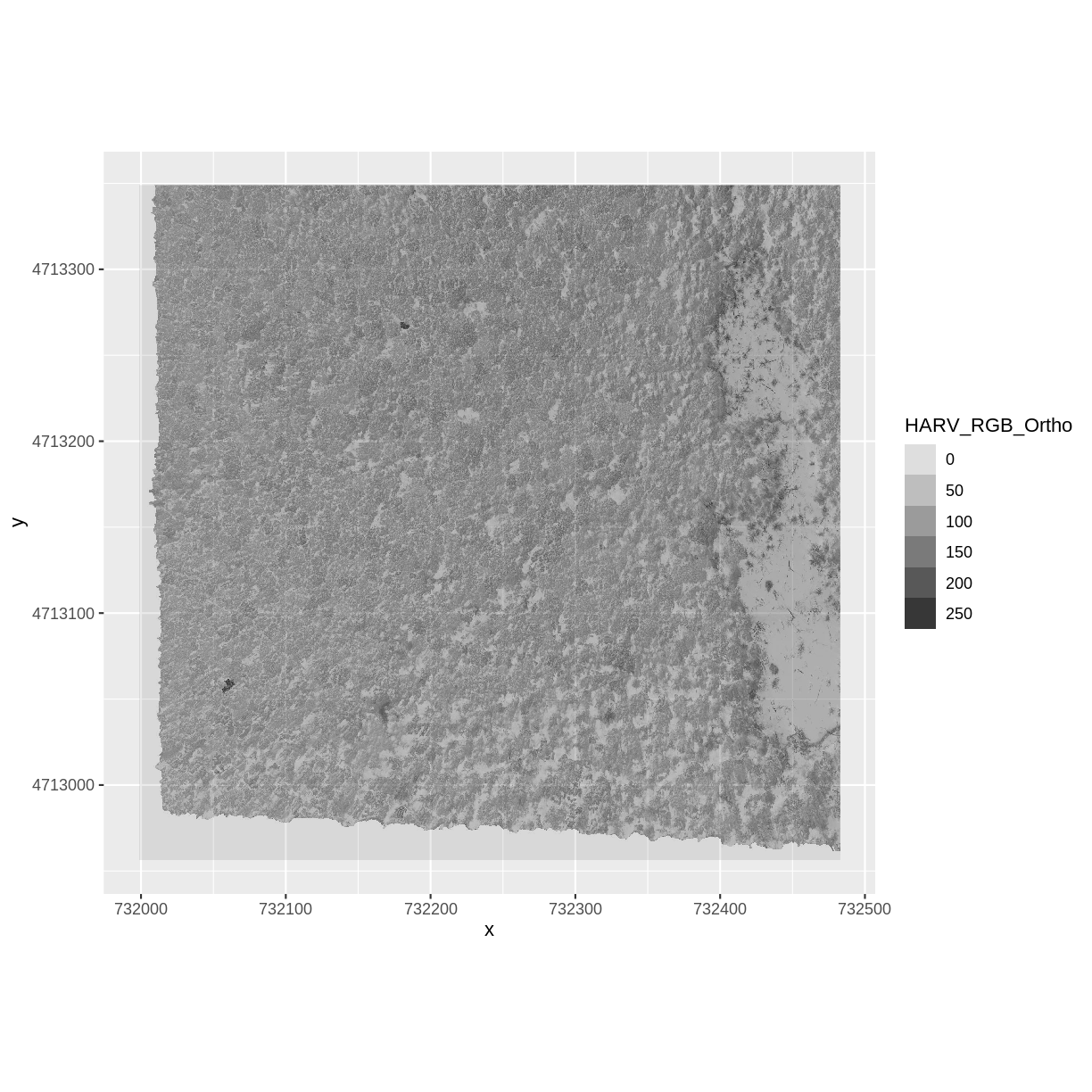## Challenge

View the attributes of this band. What are its dimensions, CRS, resolution, min and max values, and band number?

## Solution

``````RGB_band1_HARV
``````
``````class      : RasterLayer
band       : 1  (of  3  bands)
dimensions : 1571, 1938, 3044598  (nrow, ncol, ncell)
resolution : 0.25, 0.25  (x, y)
extent     : 731998.5, 732483, 4712956, 4713349  (xmin, xmax, ymin, ymax)
crs        : +proj=utm +zone=18 +datum=WGS84 +units=m +no_defs +ellps=WGS84 +towgs84=0,0,0
source     : /home/travis/build/UW-Madison-DataScience/r-raster-vector-geospatial/_episodes_rmd/data/raster/HARV_RGB_Ortho.tif
names      : HARV_RGB_Ortho
values     : 0, 255  (min, max)
``````

Notice that when we look at the attributes of this band, we see: `band: 1 (of 3 bands)`

This is R telling us that this particular raster object has more bands (3) associated with it.

## Data Tip

The number of bands associated with a raster object can also be determined using the `nbands()` function: syntax is `nbands(RGB_band1_HARV)`.

### Image Raster Data Values

As we saw in the previous exercise, this raster contains values between 0 and 255. These values represent degrees of brightness associated with the image band. In the case of a RGB image (red, green and blue), band 1 is the red band. When we plot the red band, larger numbers (towards 255) represent pixels with more red in them (a strong red reflection). Smaller numbers (towards 0) represent pixels with less red in them (less red was reflected). To plot an RGB image, we mix red + green + blue values into one single color to create a full color image - similar to the color image a digital camera creates.

### Import A Specific Band

We can use the `raster()` function to import specific bands in our raster object by specifying which band we want with `band = N` (N represents the band number we want to work with). To import the green band, we would use `band = 2`.

``````RGB_band2_HARV <-  raster("data/raster/HARV_RGB_Ortho.tif", band = 2)
``````

We can convert this data to a data frame and plot the same way we plotted the red band:

``````RGB_band2_HARV_df <- as.data.frame(RGB_band2_HARV, xy = TRUE)
``````
``````ggplot() +
geom_raster(data = RGB_band2_HARV_df,
aes(x = x, y = y, alpha = HARV_RGB_Ortho)) +
coord_equal()
``````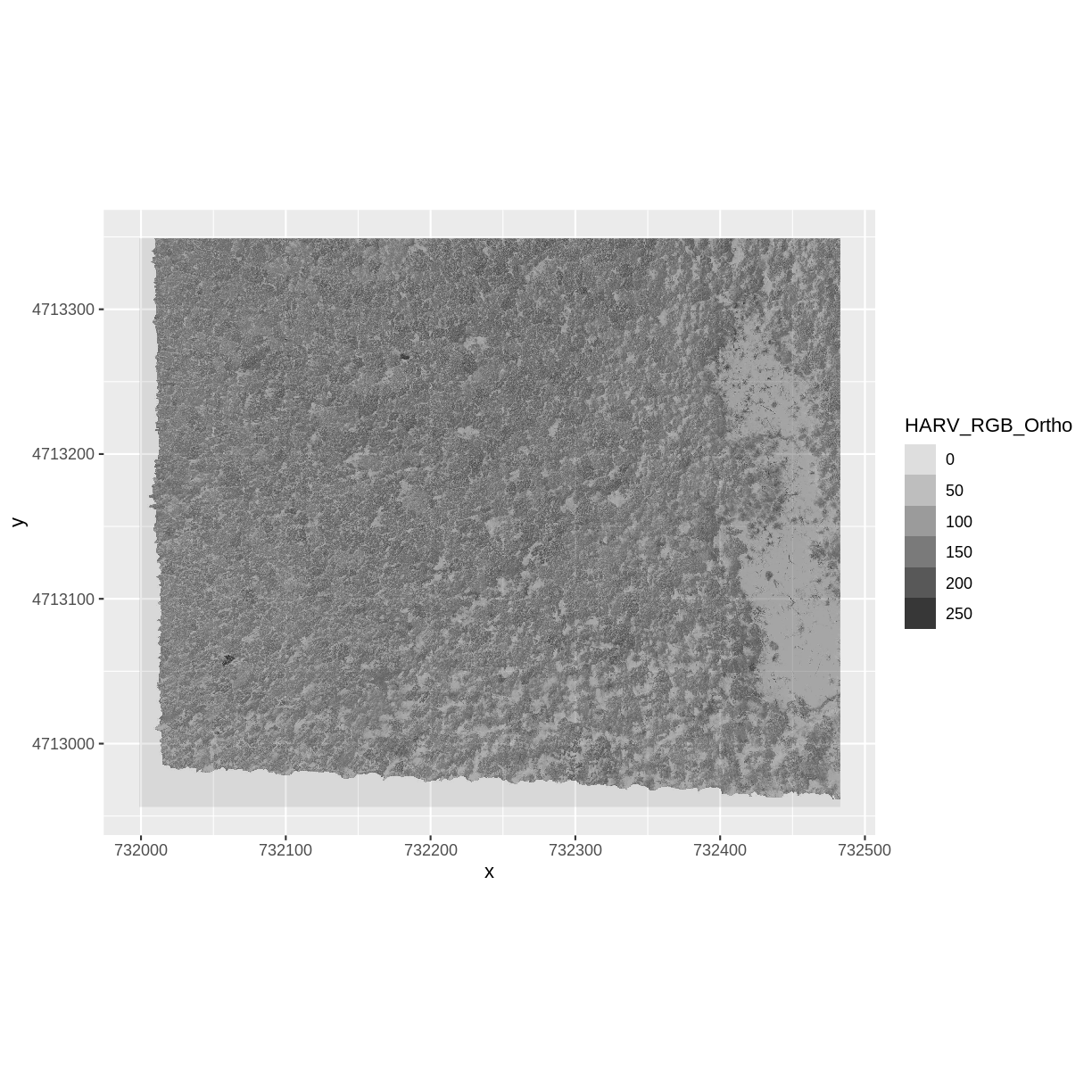## Raster Stacks in R

Next, we will work with all three image bands (red, green and blue) as an R RasterStack object. We will then plot a 3-band composite, or full color, image.

To bring in all bands of a multi-band raster, we use the`stack()` function.

``````RGB_stack_HARV <- stack("data/raster/HARV_RGB_Ortho.tif")
``````

Let’s preview the attributes of our stack object:

``````RGB_stack_HARV
``````
``````class      : RasterStack
dimensions : 1571, 1938, 3044598, 3  (nrow, ncol, ncell, nlayers)
resolution : 0.25, 0.25  (x, y)
extent     : 731998.5, 732483, 4712956, 4713349  (xmin, xmax, ymin, ymax)
crs        : +proj=utm +zone=18 +datum=WGS84 +units=m +no_defs +ellps=WGS84 +towgs84=0,0,0
names      : HARV_RGB_Ortho.1, HARV_RGB_Ortho.2, HARV_RGB_Ortho.3
min values :                0,                0,                0
max values :              255,              255,              255
``````

We can view the attributes of each band in the stack in a single output:

``````RGB_stack_HARV@layers
``````
``````[]
class      : RasterLayer
band       : 1  (of  3  bands)
dimensions : 1571, 1938, 3044598  (nrow, ncol, ncell)
resolution : 0.25, 0.25  (x, y)
extent     : 731998.5, 732483, 4712956, 4713349  (xmin, xmax, ymin, ymax)
crs        : +proj=utm +zone=18 +datum=WGS84 +units=m +no_defs +ellps=WGS84 +towgs84=0,0,0
source     : /home/travis/build/UW-Madison-DataScience/r-raster-vector-geospatial/_episodes_rmd/data/raster/HARV_RGB_Ortho.tif
names      : HARV_RGB_Ortho.1
values     : 0, 255  (min, max)

[]
class      : RasterLayer
band       : 2  (of  3  bands)
dimensions : 1571, 1938, 3044598  (nrow, ncol, ncell)
resolution : 0.25, 0.25  (x, y)
extent     : 731998.5, 732483, 4712956, 4713349  (xmin, xmax, ymin, ymax)
crs        : +proj=utm +zone=18 +datum=WGS84 +units=m +no_defs +ellps=WGS84 +towgs84=0,0,0
source     : /home/travis/build/UW-Madison-DataScience/r-raster-vector-geospatial/_episodes_rmd/data/raster/HARV_RGB_Ortho.tif
names      : HARV_RGB_Ortho.2
values     : 0, 255  (min, max)

[]
class      : RasterLayer
band       : 3  (of  3  bands)
dimensions : 1571, 1938, 3044598  (nrow, ncol, ncell)
resolution : 0.25, 0.25  (x, y)
extent     : 731998.5, 732483, 4712956, 4713349  (xmin, xmax, ymin, ymax)
crs        : +proj=utm +zone=18 +datum=WGS84 +units=m +no_defs +ellps=WGS84 +towgs84=0,0,0
source     : /home/travis/build/UW-Madison-DataScience/r-raster-vector-geospatial/_episodes_rmd/data/raster/HARV_RGB_Ortho.tif
names      : HARV_RGB_Ortho.3
values     : 0, 255  (min, max)
``````

If we have hundreds of bands, we can specify which band we’d like to view attributes for using an index value:

``````RGB_stack_HARV[]
``````
``````class      : RasterLayer
band       : 2  (of  3  bands)
dimensions : 1571, 1938, 3044598  (nrow, ncol, ncell)
resolution : 0.25, 0.25  (x, y)
extent     : 731998.5, 732483, 4712956, 4713349  (xmin, xmax, ymin, ymax)
crs        : +proj=utm +zone=18 +datum=WGS84 +units=m +no_defs +ellps=WGS84 +towgs84=0,0,0
source     : /home/travis/build/UW-Madison-DataScience/r-raster-vector-geospatial/_episodes_rmd/data/raster/HARV_RGB_Ortho.tif
names      : HARV_RGB_Ortho.2
values     : 0, 255  (min, max)
``````

We can also use the `ggplot` functions to plot the data in any layer of our RasterStack object. Remember, we need to convert to a data frame first.

``````RGB_stack_HARV_df  <- as.data.frame(RGB_stack_HARV, xy = TRUE)
``````

Each band in our RasterStack gets its own column in the data frame. Thus we have:

``````str(RGB_stack_HARV_df)
``````
``````'data.frame':	3044598 obs. of  5 variables:
\$ x               : num  731999 731999 731999 731999 732000 ...
\$ y               : num  4713349 4713349 4713349 4713349 4713349 ...
\$ HARV_RGB_Ortho.1: num  0 0 0 0 0 0 0 0 0 0 ...
\$ HARV_RGB_Ortho.2: num  0 0 0 0 0 0 0 0 0 0 ...
\$ HARV_RGB_Ortho.3: num  0 0 0 0 0 0 0 0 0 0 ...
``````

Let’s create a histogram of the first band:

``````ggplot() +
geom_histogram(data = RGB_stack_HARV_df, aes(HARV_RGB_Ortho.1))
``````
```````stat_bin()` using `bins = 30`. Pick better value with `binwidth`.
``````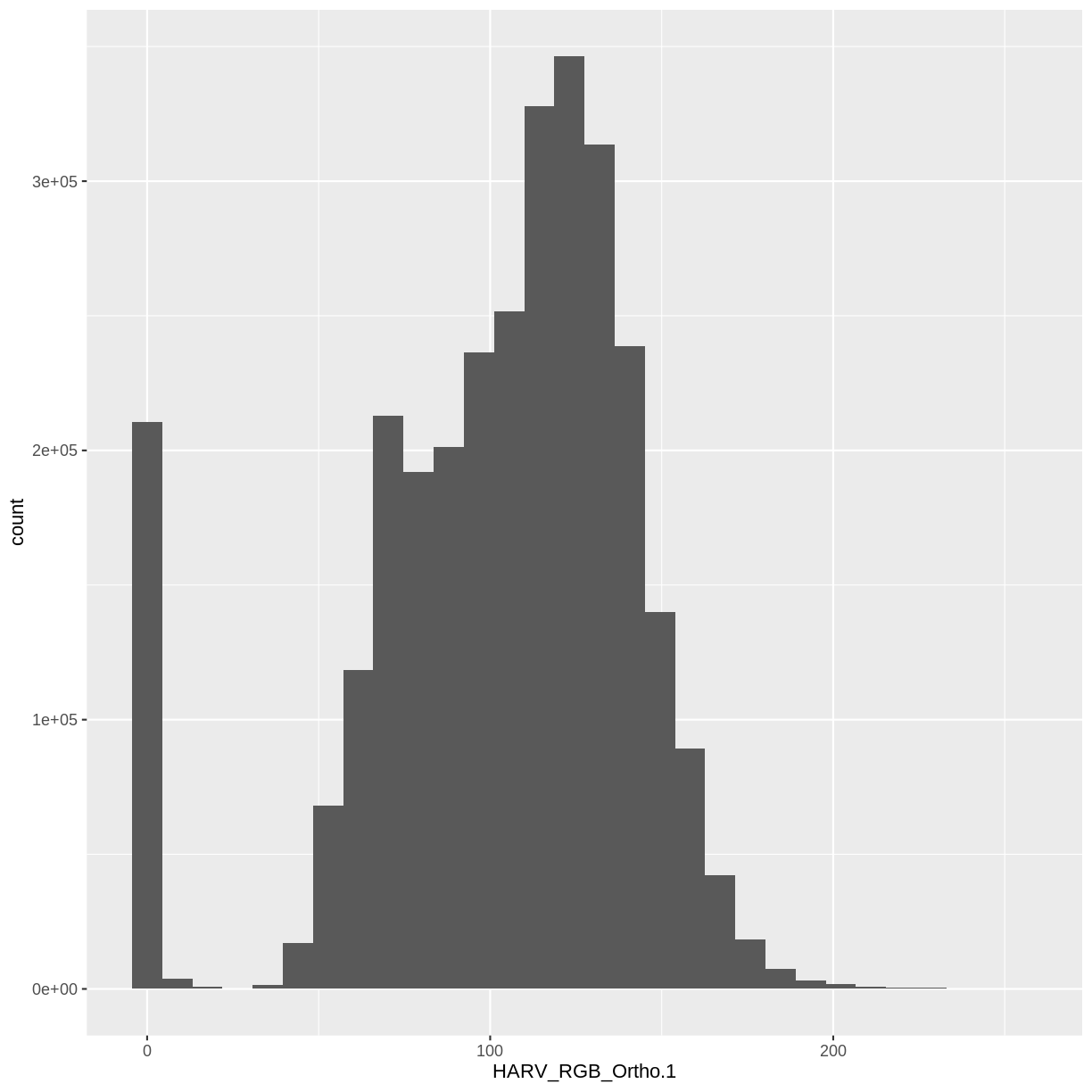And a raster plot of the second band:

``````ggplot() +
geom_raster(data = RGB_stack_HARV_df,
aes(x = x, y = y, alpha = HARV_RGB_Ortho.2)) +
coord_quickmap()
``````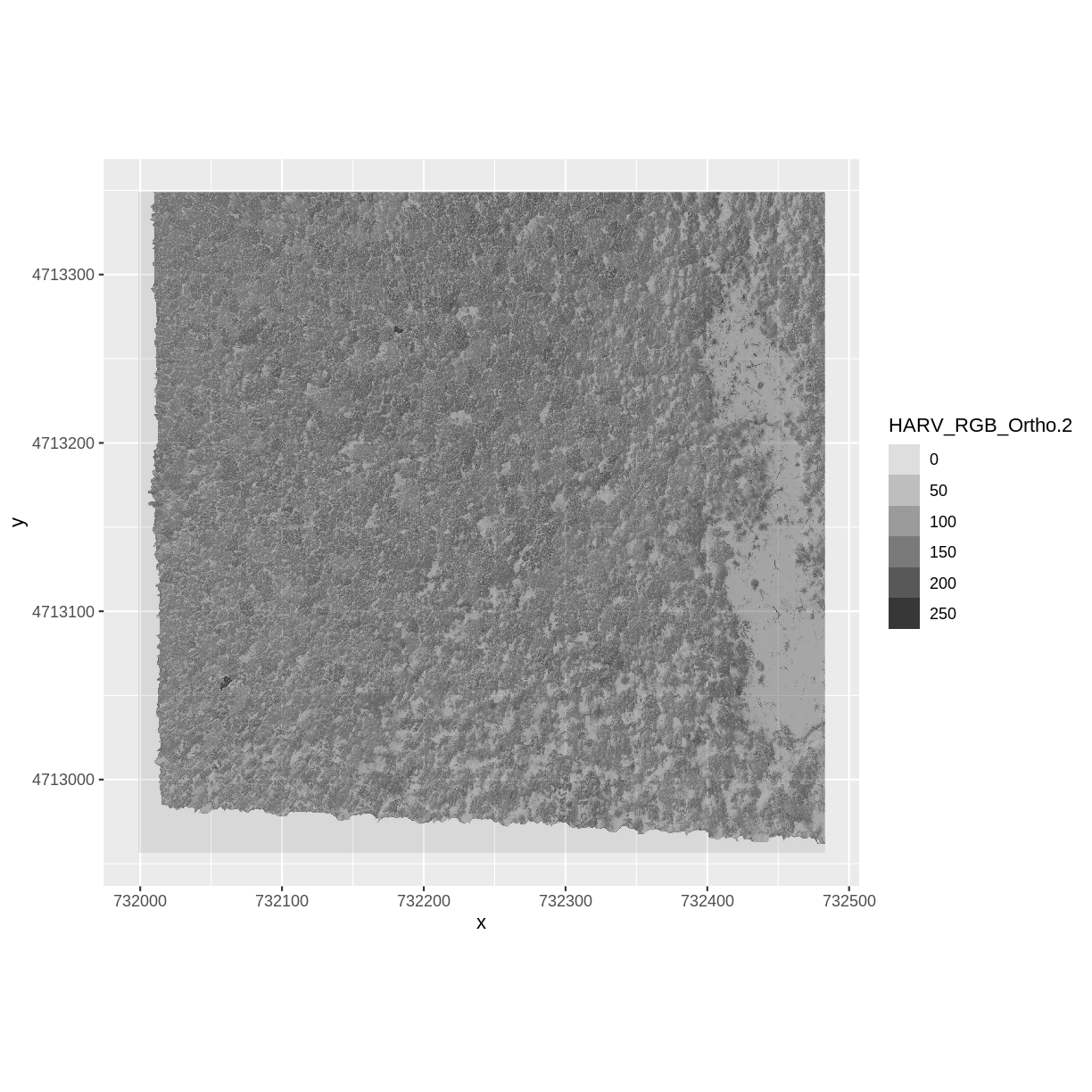We can access any individual band in the same way.

### Create A Three Band Image

To render a final three band, colored image in R, we use the `plotRGB()` function.

This function allows us to:

1. Identify what bands we want to render in the red, green and blue regions. The `plotRGB()` function defaults to a 1=red, 2=green, and 3=blue band order. However, you can define what bands you’d like to plot manually. Manual definition of bands is useful if you have, for example a near-infrared band and want to create a color infrared image.
2. Adjust the `stretch` of the image to increase or decrease contrast.

Let’s plot our 3-band image. Note that we can use the `plotRGB()` function directly with our RasterStack object (we don’t need a dataframe as this function isn’t part of the `ggplot2` package).

``````plotRGB(RGB_stack_HARV,
r = 1, g = 2, b = 3)
``````The image above looks pretty good. We can explore whether applying a stretch to the image might improve clarity and contrast by specifying `stretch="lin"` or `stretch="hist"`.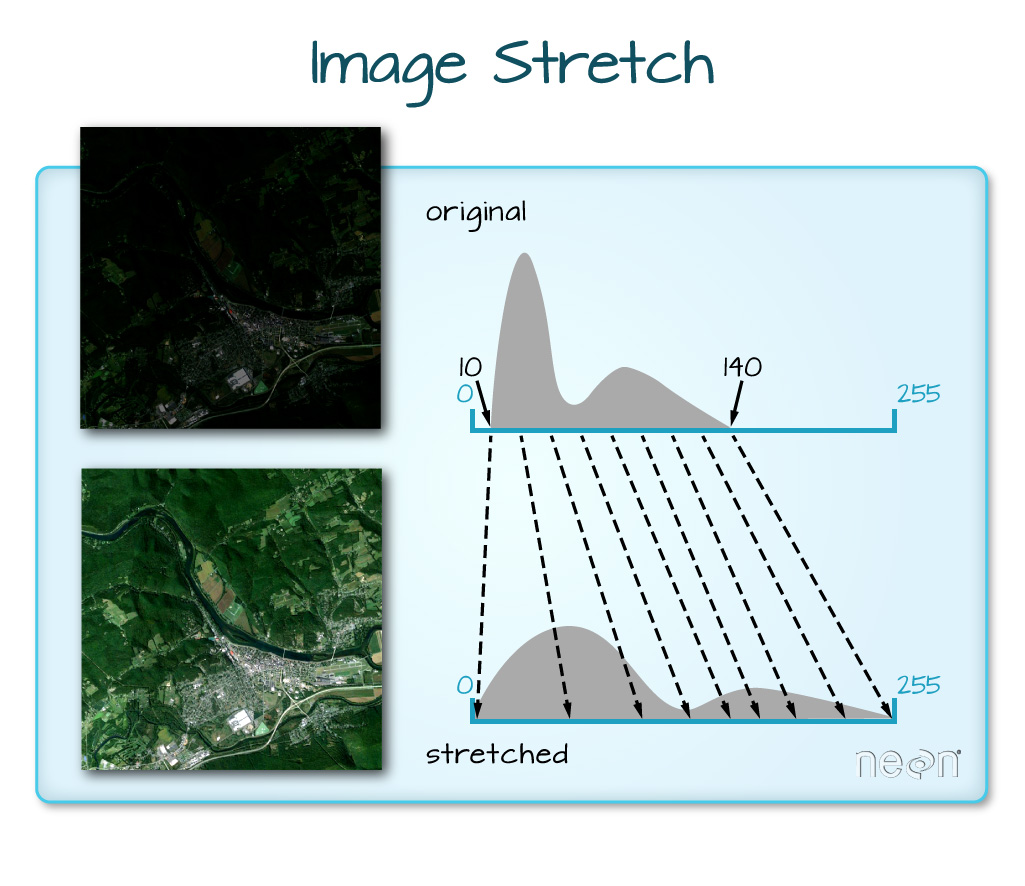When the range of pixel brightness values is closer to 0, a darker image is rendered by default. We can stretch the values to extend to the full 0-255 range of potential values to increase the visual contrast of the image.When the range of pixel brightness values is closer to 255, a lighter image is rendered by default. We can stretch the values to extend to the full 0-255 range of potential values to increase the visual contrast of the image.

``````plotRGB(RGB_stack_HARV,
r = 1, g = 2, b = 3,
scale = 800,
stretch = "lin")
``````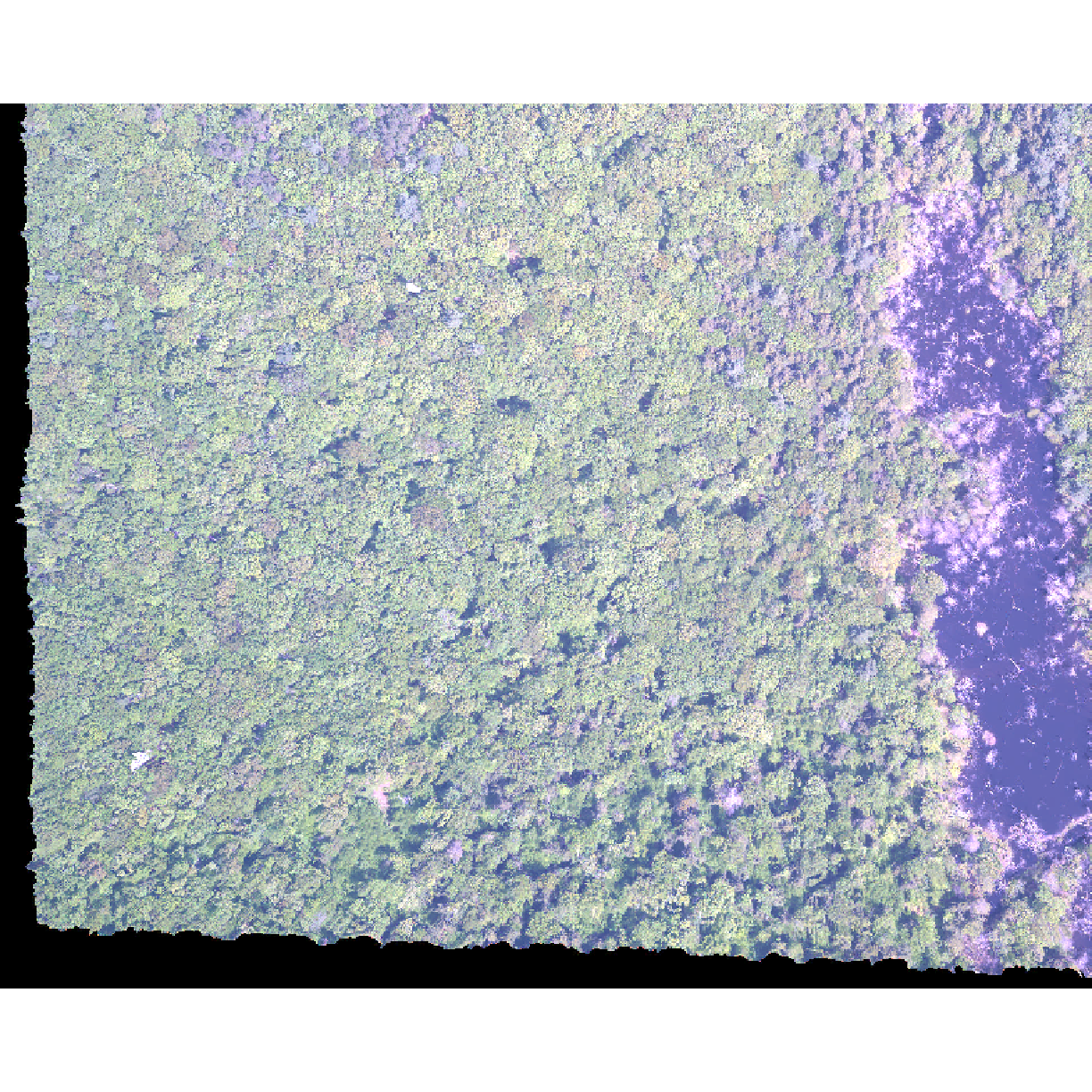``````plotRGB(RGB_stack_HARV,
r = 1, g = 2, b = 3,
scale = 800,
stretch = "hist")
``````In this case, the stretch doesn’t enhance the contrast our image significantly given the distribution of reflectance (or brightness) values is distributed well between 0 and 255.

## Challenge - NoData Values

Let’s explore what happens with NoData values when working with RasterStack objects and using the `plotRGB()` function. We will use the `HARV_Ortho_wNA.tif` GeoTIFF file in the `data/raster/` directory.

1. View the files attributes. Are there `NoData` values assigned for this file?
2. If so, what is the `NoData` Value?
3. How many bands does it have?
4. Load the multi-band raster file into R.
5. Plot the object as a true color image.
6. What happened to the black edges in the data?
7. What does this tell us about the difference in the data structure between `HARV_Ortho_wNA.tif` and `HARV_RGB_Ortho.tif` (R object `RGB_stack`). How can you check?

## Answers

1) First we use the `GDALinfo()` function to view the data attributes.

``````GDALinfo("data/raster/HARV_Ortho_wNA.tif")
``````
``````Warning in GDALinfo("data/raster/HARV_Ortho_wNA.tif"): statistics not supported
by this driver
Warning in GDALinfo("data/raster/HARV_Ortho_wNA.tif"): statistics not supported
by this driver
Warning in GDALinfo("data/raster/HARV_Ortho_wNA.tif"): statistics not supported
by this driver
``````
``````rows        1692
columns     1973
bands       3
lower left origin.x        731989.8
lower left origin.y        4712926
res.x       0.25
res.y       0.25
ysign       -1
oblique.x   0
oblique.y   0
driver      GTiff
projection  +proj=utm +zone=18 +datum=WGS84 +units=m +no_defs
file        data/raster/HARV_Ortho_wNA.tif
apparent band summary:
GDType hasNoDataValue NoDataValue blockSize1 blockSize2
1 Float32           TRUE       -9999          1       1973
2 Float32           TRUE       -9999          1       1973
3 Float32           TRUE       -9999          1       1973
apparent band statistics:
Bmin       Bmax Bmean Bsd
1 -4294967295 4294967295    NA  NA
2 -4294967295 4294967295    NA  NA
3 -4294967295 4294967295    NA  NA
Metadata:
AREA_OR_POINT=Area
``````

2) From the output above, we see that there are `NoData` values and they are assigned the value of -9999.

3) The data has three bands.

4) To read in the file, we will use the `stack()` function:

``````HARV_NA <- stack("data/raster/HARV_Ortho_wNA.tif")
``````

5) We can plot the data with the `plotRGB()` function:

``````plotRGB(HARV_NA,
r = 1, g = 2, b = 3)
``````6) The black edges are not plotted. 7) Both data sets have `NoData` values, however, in the RGB_stack the NoData value is not defined in the tiff tags, thus R renders them as black as the reflectance values are 0. The black edges in the other file are defined as -9999 and R renders them as NA.

``````GDALinfo("data/raster/HARV_RGB_Ortho.tif")
``````
``````rows        1571
columns     1938
bands       3
lower left origin.x        731998.5
lower left origin.y        4712956
res.x       0.25
res.y       0.25
ysign       -1
oblique.x   0
oblique.y   0
driver      GTiff
projection  +proj=utm +zone=18 +datum=WGS84 +units=m +no_defs
file        data/raster/HARV_RGB_Ortho.tif
apparent band summary:
GDType hasNoDataValue NoDataValue blockSize1 blockSize2
1 Float32           TRUE    -3.4e+38          1       1938
2 Float32           TRUE    -3.4e+38          1       1938
3 Float32           TRUE    -3.4e+38          1       1938
apparent band statistics:
Bmin Bmax    Bmean      Bsd
1    0  255 102.4938 39.89983
2    0  255 123.6979 45.93316
3    0  255  90.4154 28.54954
Metadata:
AREA_OR_POINT=Area
``````

## Data Tip

We can create a RasterStack from several, individual single-band GeoTIFFs too. We will do this in a later episode, Raster Time Series Data in R.

## RasterStack vs RasterBrick in R

The R RasterStack and RasterBrick object types can both store multiple bands. However, how they store each band is different. The bands in a RasterStack are stored as links to raster data that is located somewhere on our computer. A RasterBrick contains all of the objects stored within the actual R object. In most cases, we can work with a RasterBrick in the same way we might work with a RasterStack. However a RasterBrick is often more efficient and faster to process - which is important when working with larger files.

## More Resources

You can read the help for the `brick()` function by typing `?brick`.

We can turn a RasterStack into a RasterBrick in R by using `brick(StackName)`. Let’s use the `object.size()` function to compare RasterStack and RasterBrick objects. First we will check the size of our RasterStack object:

``````object.size(RGB_stack_HARV)
``````
``````44248 bytes
``````

Now we will create a RasterBrick object from our RasterStack data and view its size:

``````RGB_brick_HARV <- brick(RGB_stack_HARV)

object.size(RGB_brick_HARV)
``````
``````73084136 bytes
``````

Notice that in the RasterBrick, all of the bands are stored within the actual object. Thus, the RasterBrick object size is much larger than the RasterStack object.

You use the `plotRGB()` function to plot a RasterBrick too:

``````plotRGB(RGB_brick_HARV)
``````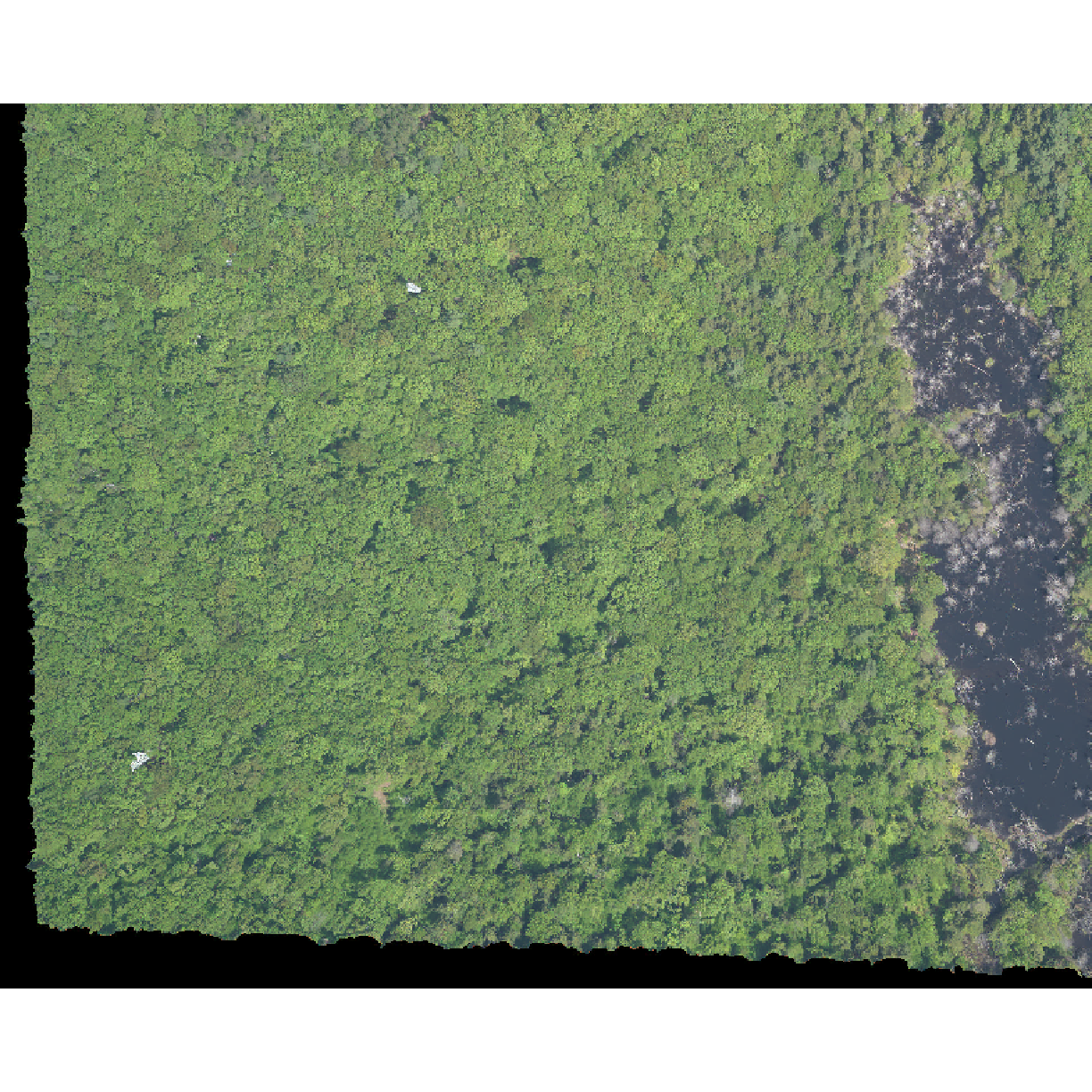## Challenge: What Functions Can Be Used on an R Object of a particular class?

We can view various functions (or methods) available to use on an R object with `methods(class=class(objectNameHere))`. Use this to figure out:

1. What methods can be used on the `RGB_stack_HARV` object?
2. What methods can be used on a single band within `RGB_stack_HARV`?
3. Why do you think there is a difference?

## Answers

1) We can see a list of all of the methods available for our RasterStack object:

``````methods(class=class(RGB_stack_HARV))
``````
``````   !                     !=                    [
 [[                    [[<-                  [<-
 %in%                  ==                    \$
 \$<-                   addLayer              adjacent
 aggregate             all.equal             animate
 approxNA              area                  Arith
 as.array              as.character          as.data.frame
 as.integer            as.list               as.logical
 as.matrix             as.vector             atan2
 bbox                  boxplot               brick
 calc                  cellFromRowCol        cellFromRowColCombine
 cellFromXY            cellStats             clamp
 click                 coerce                colFromCell
 colFromX              colSums               Compare
 coordinates           corLocal              couldBeLonLat
 cover                 crop                  crosstab
 crs<-                 cut                   cv
 density               dim                   dim<-
 disaggregate          dropLayer             extend
 extent                extract               flip
 freq                  getValues             getValuesBlock
 getValuesFocal        hasValues             head
 hist                  image                 init
 interpolate           intersect             is.factor
 is.finite             is.infinite           is.na
 is.nan                isLonLat              KML
 labels                length                levels
 levels<-              log                   Logic
 mask                  match                 Math
 Math2                 maxValue              mean
 merge                 minValue              modal
 mosaic                names                 names<-
 ncell                 ncol                  ncol<-
 nlayers               nrow                  nrow<-
 origin                origin<-              overlay
 pairs                 persp                 plot
 plotRGB               predict               print
 proj4string           proj4string<-         quantile
 raster                rasterize             ratify
 readAll               readStart             readStop
 reclassify            rectify               res
 res<-                 resample              rotate
 rowColFromCell        rowFromCell           rowFromY
 rowSums               sampleRandom          sampleRegular
 scale                 select                setMinMax
 setValues             shift                 show
 spplot                stack                 stackSelect
 stretch               subs                  subset
 Summary               summary               t
 tail                  text                  trim
 unique                unstack               values
 values<-              weighted.mean         which.max
 which.min             whiches.max           whiches.min
 wkt                   writeRaster           xFromCell
 xFromCol              xmax                  xmax<-
 xmin                  xmin<-                xres
 xyFromCell            yFromCell             yFromRow
 ymax                  ymax<-                ymin
 ymin<-                yres                  zonal
 zoom
see '?methods' for accessing help and source code
``````

2) And compare that with the methods available for a single band:

``````methods(class=class(RGB_stack_HARV))
``````
``````Warning in .S3methods(generic.function, class, envir): 'class' is of length > 1;
only the first element will be used
``````
``````  [             [<-           anyDuplicated as_tibble     as.data.frame
 as.raster     bind          boxplot       brick         coerce
 coordinates   determinant   duplicated    edit          extent
 extract       head          initialize    isSymmetric   Math
 Math2         Ops           raster        rasterize     relist
 subset        summary       surfaceArea   tail          trim
 unique        weighted.mean writeValues
see '?methods' for accessing help and source code
``````

3) There are far more things one could or want to ask of a full stack than of a single band.

## Key Points

• A single raster file can contain multiple bands or layers.

• Use the `stack()` function to load all bands in a multi-layer raster file into R.

• Individual bands within a stack can be accessed, analyzed, and visualized using the same functions as single bands.